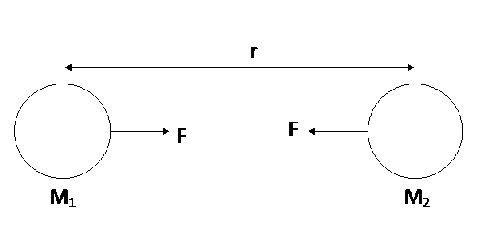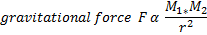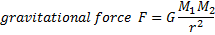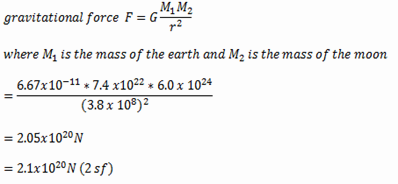Custom Search
If you want to discuss more on this or other issues related to Physics, feel free to leave a message on my Facebook page.

## Wednesday, March 10, 2010

### what is the gravitational force?

The gravitational force is an attractive force that a mass exerts on another mass when the former is placed in the latter’s gravitational field.

Fig 1

In fig 1 above we have two masses m1 and m2 that are situated at a distance r apart. As you know these two masses will create gravitational fields around themselves such that mass M1 will exert a gravitational force F2 on mass M2 while the mass M2 will exert a gravitational force F1 on mass M1. That is each mass will exert a gravitational force on the other mass.

And from the third law of motion we know that “For every action there is an equal and opposite reaction.

Hence the gravitational force F2 will be equal to the gravitational force F1 .

F1  = F2

If the two forces are equal then they have the same magnitude and as a result

|F1 | = |F2 | = F

We can thus replace F1 and  F2  by F as shown in fig 2 below.Fig 2

Law of gravitation

In order to calculate the magnitude of the force that each mass will exert on the other the law of gravitation must be used.

It merely states that the gravitational force that the two objects will exert on each other is directly proportional to the product of the two masses and inversely proportional to the square of their distance of separation.

Simply written in an equation it will be as shown below:Removing the proportionality sign you will obtain the equation below:Where G is the universal gravitational constant

G = 6.67 x 10-11N m2 kg-2

Example 1

The moon and the earth are separated by a distance of 3.8x 108 m. The mass of the moon is 6.4 x 1022 kg while that of the earth is 6.0x1024 kg. Calculate the gravitational force between the moon.